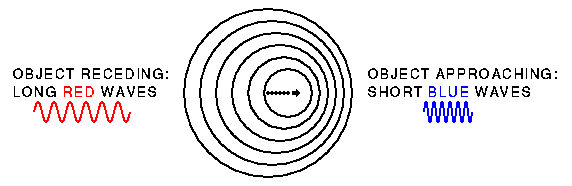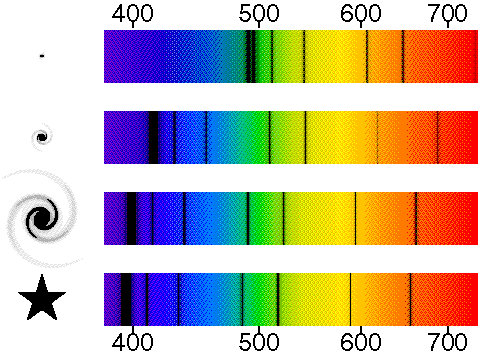# Doppler Shift

## Red and blue shifts

Light from moving objects will appear to have different wavelengths depending on the relative motion of the source and the observer.Observers looking at an object that is moving away from them see light that has a longer wavelength than it had when it was emitted (a redshift), while observers looking at an approaching source see light that is shifted to shorter wavelength (a blueshift).

The schematic diagram below shows a galactic star at the bottom left with its spectrum on the bottom right. The spectrum shows the dark absorption lines first seen by Fraunhofer. These lines can be used to identify the chemical elements in distant stars, but they also tell us the radial velocity. The other three spectra and pictures from bottom to top show a nearby galaxy, a medium distance galaxy, and a distant galaxy. The pictures on the left are negatives, of course, so the brightest parts of the galaxies are black. Notice how the pattern of absorption lines shifts to the red as the galaxies get fainter. The numbers above and below the spectra are the measured wavelengths in nm [nanometers].In the star which is at rest with respect to us, or in a laboratory standard, the line wavelengths are 393 & 397 nm from Ca II [ionized calcium]; 410, 434, 486 & 656 nm from H I [atomic hydrogen]; 518 nm from Mg I [neutral magnesium]; and 589 nm from Na I [neutral sodium]. By measuring the amount of the shift to the red, we can determine that the bright galaxy is moving away at 3,000 km/sec, which is 1 percent of the speed of light, because its lines are shifted in wavelength by 1 percent to the red. The redshift z is defined such that:
```      lambda(observed)
1+z = ----------------
lambda(emitted)
```
which is
```      397   401   414   438   491   523   595   663
1+z = --- = --- = --- = --- = --- = --- = --- = --- = 1.01
393   397   410   434   486   518   589   656
```
in this case so z = 0.01 for the bright galaxy. The radial velocity is usually approximated by v(rad) = cz, where c is the speed of light, The medium bright galaxy is moving away at 15,000 km/sec with z = 0.05, while the faintest and most distant galaxy is moving away at 75,000 km/sec with z = 0.25. When z is larger than 1 then cz is faster than the speed of light and, while recession velocities faster than light are allowed, this approximation using cz as the recession velocity of an object is no longer valid. Thus for the largest known redshift of z=6.3, the recession velocity is not 6.3*c = 1,890,000 km/sec. It is also not the 285,254 km/sec given by the special relativistic Doppler formula 1+z = sqrt((1+v/c)/(1-v/c)). The actual recession velocity for this object depends on the cosmological parameters, but for an OmegaM=0.3 vacuum-dominated flat model the velocity is 585,611 km/sec. This is faster than light.

Tutorial: Part 1 | Part 2 | Part 3 | Part 4
FAQ | Age | Distances | Bibliography | Relativity